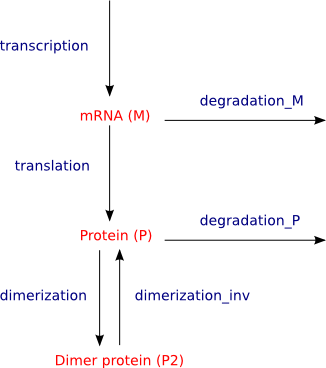# An illustrative example

In order to see how we can model a biochemical network in Bio-PEPA, we consider a simple genetic network with a negative feedback through dimers. This is from the paper Fluctuations and Slow Variables in Genetic Networks, by Bundschuh and co-authors.The model is composed of three biological species:

• the mRNA molecule (M),
• the protein in monomer form (P),
• the protein in dimeric form (P2).

These species are involved in five interactions:

• the transcription of the mRNA from the genes/DNA, with the inhibition by means of P2 (transcription),
• the translation of the protein P from M (translation),
• the dimerization of P (dimerization),
• the inverse process of dimerization (dimerization_inv).

.

The species of the network are represented by the following species components in Bio-PEPA:

``` M  = (transcription,1) >> M + (translation,1) << M + (degradation_M,1) << M; P  = (translation,1) >> P + (degradation_P,1) << P + (dimerization,2) << P +     (dimerization_inv,2) >> P; P2 = (transcription,1) (-) P2 + (dimerization,1) >> P2 + (dimerization_inv,1) << P; ```

The model component of the Bio-PEPA system corresponding to the network above is:

``` M(0) <translation > P(0) <dimerization, dimerization_inv > P2(0) ```

The mapping is based on the following abstraction:

• Each reaction is represented by an action type;

• Each species is represented by a species component. Each sub-term represents one of the reactions in which the species is involved. For each sub-term we consider the stoichiometric coefficient and the role of the species with respect to the reaction, represented by a specific symbol: >> stands for product, << for reactant, (+) for enzyme and (-) for inhibitor. Note that these symbols are the ones used in the syntax of the Bio-PEPA workbench; more formally the Bio-PEPA notation is ↓ for <<, ↑ for >>, ⊕ for (+) and ⊖ for (-).
Consider the protein P in the definitions above. It is involved in four reactions with different roles and stoichiometry: in the translation, P is a product and has stoichiometry one, in the degradation it is a reactant and has stoichiometry one, in the dimerization it is a reactant and has stoichiometry two and finally in the inverse of dimerization it is a product and has stoichiometry two.

• The model component describes which species are involved in the system and their initial quantity (in the example above the initial quantity of each species is set to zero).

In addition to these elements, there is the definition of the functional rates, expressing the kinetic laws associated with each reaction, and the definition of parameter constants:

``` f_transcription = (v • D)/(KM + P2);   f_translation = k2 • M;   f_degradation_M = k3 • M;   f_degradation_P = k4 • P;   f_dimerization = k5 • P • P;   f_monomerization = k5i • P2; ``` ``` KM = 356 molecules;   v = 2.19 s-1;   k2 = 0.043 s-1;   k3 = 0.039 s-1;   k4 = 0.0007 s-1;   k5 = 0.025 s-1;   k5i = 0.5 s-1; ```

Note that all the kinetic laws are of kind mass-action with the exception of the first, that expresses inhibition.

Finally, we have the definition of the compartment where the species are and some information about levels, step size, etc. to use in the analysis.

For details about the model see the reference paper.